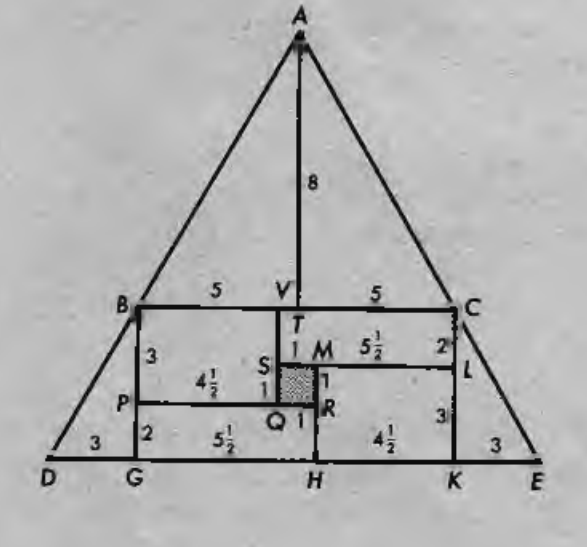# Are you smarter than an old book?

Geometry Level 3In the figure above there are 4 rectangles, 4 right triangles, and a square hole.

a) Determine the sum of the 8 areas (do not include the area of the hole).

b) Let x be the length of DE, and y the height from point A to DE. Calculate $\frac { xy }{ 2 }$.

Can you explain why parts (a) and (b) have the same result?

×